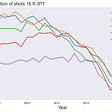# Outliers: Meaning and Method To Find Them in Data.

An outlier is an observation that lies an abnormal distance from other values in a random sample from a population.

Causes of outlier.

The following are common of the causes of the existence of outlier in a data set:

1. Data Entry Error - Human Error such as errors caused during data collection, recording, or entry.
2. Data Processing Error - These are caused when manipulation or extraction of the data set is performed.
3. Experimental Error - These errors are caused during data extraction or experiment planning or while performing an experiment.

Effect of outlier on a data set.

Outliers have a great impact on result of data analysis and statistical measures.

Some of the most common effects are:

• If the outliers are non-randomly distributed, they can decrease normality.
• It increases the error variance and reduces the power of statistical tests.
• They can cause bias and/or influence estimates.
• They can also affect the basic assumption of regression and other statistical models.

Method Of Finding Outliers

We can detect outliers easily in a data set with the help of five-number summary, which comprises the followings:

1. Minimum
2. First Quartile (Q1)
3. Median
4. Third Quartile (Q3)
5. Maximum

Whenever we want to remove outliers, we have to define the Lower Fence and Higer Fence.

Minimum = Q1 - 1.5*IQR

Maximum = Q3+1.5*IQR

IQR (Interquartile Range) = Q3 - Q1

Q1 = 25%

Q3 = 75%

Let’s consider this data.

1, 2,2,2,3,3,4,5,5,5,6,6,6,6,7,8, 8, 9, 27

Step1

Find the value exists at Q1 = 25% and also Q3 = 75%

value = (percentile/100 ) * (n + 1)

n = 19

Q1 value= (25/100) * (19 + 1) th

Q1 value = 5 th

Steps2

Using the position number to find the value corresponds to it.

value: 1, 2,2,2,3,3,4,5,5,5,6,6,6,6,7,8, 8, 9, 27

Now take the value that likely fall in index of 5th

Therefore, Q1 value = 3

Q3 value= (75/100) * (19 + 1) th

Q3 value = 15th

Now take the value that likely fall in index of 15th

Therefore, Q3 value = 7

IQR = Q3 - Q1

IQR = 7 - 3

IQR = 4

Minimum = Q1–1.5*IQR

Minimum = 3 - 1.5 * 4

Minimum = -3

Maximum = Q3+1.5*IQR

Maximum = 7 + 1.5 * 4

Maxinum = 13

[-3 to 13] this means that any values below -3 and higher than 13 are considered an outlier.

We can also detect outliers in a data set with the help of python programming language.

A box plot is a standardized way of displaying the distribution of data based on a five-number summary (“minimum”, first quartile (Q1), median, third quartile (Q3), and “maximum”). It can tell you about your outliers and what their values are. It can also tell you if your data is symmetrical, how tightly your data is grouped, and if and how your data is skewed.

# Import libraries
import matplotlib.pyplot as plt
import numpy as np

# Creating dataset
np.random.seed(10)
data = np.random.normal(100, 20, 200)

fig = plt.figure(figsize =(10, 7))

# Creating plot
plt.boxplot(data)

# show plot
plt.show()

Output:

The diagram let’s understand that any values below 60 and higher than 150 are considered as outliers.

--

--

--

## More from Ridwanullah Ajayi

Love podcasts or audiobooks? Learn on the go with our new app.

## How much do we have to know to predict?## Everyone Can Learn Programming Easily — If they Know English## Moving in to Lisbon## The Evolution of NBA Shot Selection## The “Violin plot”## Reshaping Pandas Dataframes Melt And Unmelt## Understanding SVMs’: For Image Classification## Building NLP datasets: the leap of faith## THE FUTURE OF TIME DATA## Breaking the Cycle of Mom Guilt — The New Governess